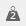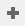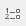# Calculating mean scores after banding a quantity variable

You are here:

The following example uses the Crocodile Rock Cafe survey supplied with Snap. Question 8 is an attitude question, asking respondents to rate their experience on a scale of 1 (poor) to 10 (excellent). It is a quantity variable, so to find out how many people entered each value, it must be banded into the different values, using a derived variable. You can then display the results as a frequency table.

You can calculate the mean scores of the banded variable by scoring it, so that the different codes in the derived variables are matched back to numeric values. Open the Crocodile Rock Cafe survey.

1. Clickto display the Weights Window.
2. Clickto add a new weight (score10) and specify the weight matrix as:
1. You can use other scoring values if you wish. For example 100, 90, 80, etc.
2. Clickto display the Variables window.
3. Clickto add a new variable.
4. Specify the Variable Details for derived variable which bands Q8 into ten codes. The example shown labels the bands as 1 to 10, but you could label them Poor to Excellent.
1. Clickto display the Analysis Definition dialog box.
2. Enter your new derived variable in the Analysis.
3. Specify the Break as Q11, age range.
4. Click the Summary Statistics tab.
5. Double-click Mean in the left-hand column to add it to the list of statistics.
6. A box appears for you to score the mean. Specify the Score as your new score (score10).
1. Click on the Base/Labels tab. Because the analysis variable has been scored, change the Analysis labels to make this obvious on the table.
2. Click Insert by the Analysis label and select {label}. This inserts the appropriate code label of the analysis variable into the table.
3. Type “scored as” into the Analysis label field.
4. Click Insert by the Analysis label and select {score}. This inserts the score for that code into the table.
1. Click OK to create the table.
Contents